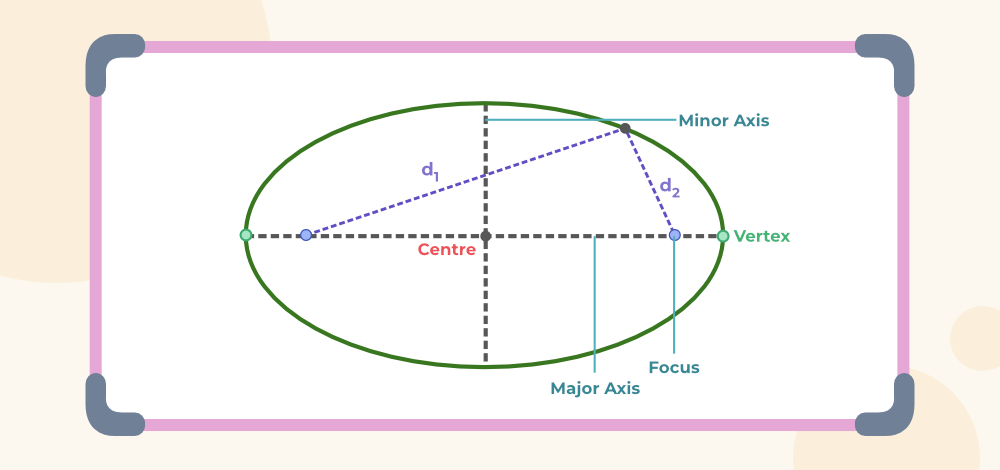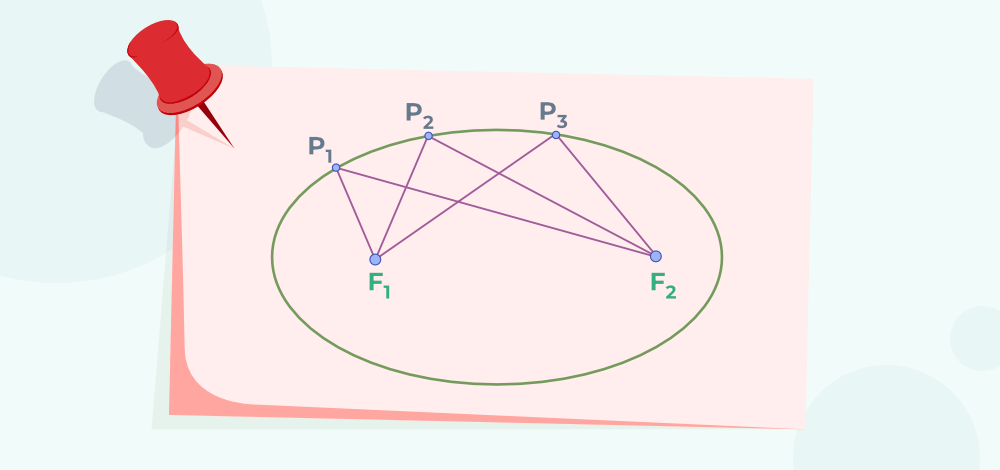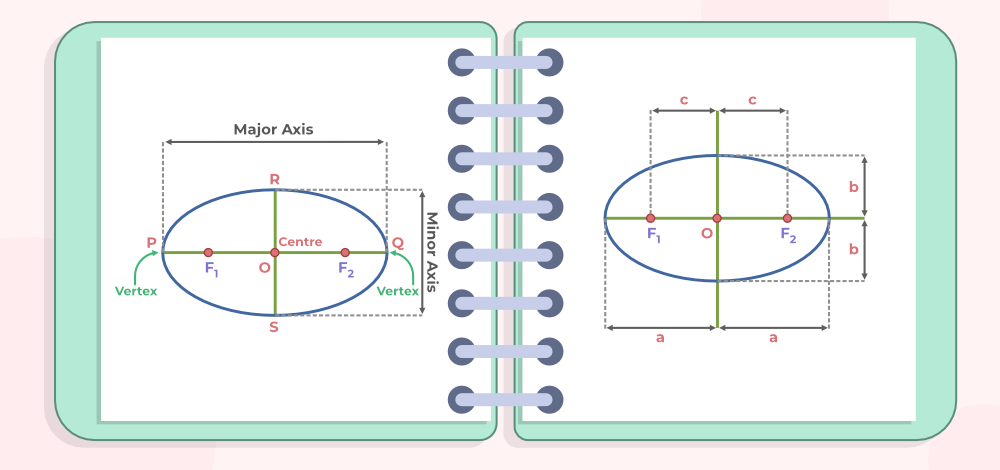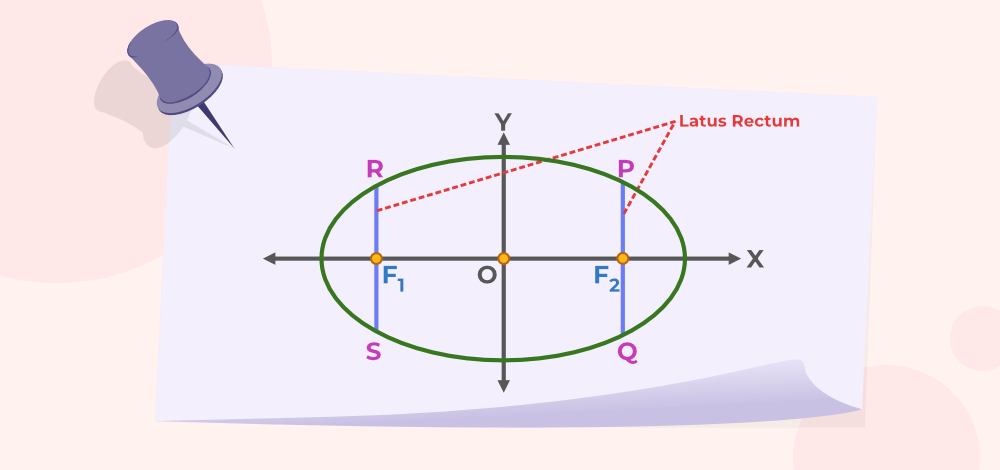Open in App
Not now

# Ellipse

• Last Updated : 19 Mar, 2023

An ellipse is the locus of all points on a plane with constant distances from two fixed points in the plane. The fixed points encircled by the curve are known as foci (singular focus). The constant ratio is the eccentricity of the ellipse and the fixed line is the directrix. In this article, we will learn about the ellipse in detail.

## What is Ellipse?

In geometry, an ellipse is a two-dimensional shape described along its axes. When a cone is intersected by a plane at an angle with respect to its base, an ellipse is created. There are two focal points. The total of the two distances to the focal point is always constant for all places along the curve. A circle is also an ellipse in which the foci are all at the same location, which is the circle’s centre.## Definition

The locus of all the points on an XY-plane, whose sum of the distance from two fixed points is constant is called an Ellipse.

Ellipse is produced, when a plane cuts the cone at an angle with the base. If the plane intersected by the cone is parallel to the base, then it forms a circle.

## Ellipse Shape

In geometry, an ellipse is a two-dimensional shape, that is defined along its axes. An ellipse is formed when a cone is intersected by a plane at an angle with respect to its base.

It has two focal points. The sum of the two distances to the focal point, for all the points in the curve, is always constant.

A circle is also an ellipse, where the foci are at the same point, which is the centre of the circle.

## Major and Minor Axis

An Ellipse has two axes along the x-axis and the y-axis that support its structure. They are called,

• Major axis
• Minor Axis

The axis with the longer diameter of the ellipse is called as major axis. It is denoted by the symbol ‘a’ while the smaller diameter axis is called the minor axis. It is denoted by the symbol ‘b’, and both axes intersect at the centre of the ellipse.

Half of the major axis is called the semi-major axis and half of the minor axis is called the semi-minor axis.

## Eccentricity of Ellipse

The eccentricity of an ellipse is defined as the ratio of distances from the centre of the ellipse to the semi-major axis of the ellipse.

e = c/a

where
c is the focal length and
a is the semi-major axis length.

The eccentricity of an ellipse is always larger than 1.

Furthermore, c2 = a2 – b2.

As a result, eccentricity becomes:

e = √[(a2 – b2)/a2]

e = √[1-(b2/a2)]

## Properties of Ellipse

Various properties of an ellipse are,

• Ellipse includes two focal points, also referred to as foci.
• A fixed distance is referred to as a directrix.
• The eccentricity of an ellipse ranges from 0 to 1. 0≤e<1
• The whole sum of each distance from an ellipse’s locus to its two focal points is constant.
• Ellipse has one major and one minor axis, as well as a centre.

## Ellipse Formula

Take a point P at one end of the major axis, as indicated. As a result, the total of the distances between point P and the foci is,

F1P + F2P = F1O + OP + F2P = c + a + (a–c) = 2a

Then, select a point Q on one end of the minor axis. The sum of the distances between Q and the foci is now,

F1Q + F2Q = √ (b2 + c2) + √ (b2 + c2) = 2√ (b2 + c2)

We already know that points P and Q are on the ellipse. As a result, by definition, we have

2√ (b2 + c2) = 2a

then √ (b2 + c2) = a

i.e. a2 = b2 + c2 or c2 = a2 – b2

The following is the equation for ellipse.

c2= a2 – b2

## Derivation of Ellipse Equation

The figure represents an ellipse such that P1F1 + P1F2 = P2F1 + P2F2 = P3F1 + P3F2 is a constant. This constant is always greater than the distance between the two foci. When both the foci are joined with the help of a line segment then the mid-point of this line segment joining the foci is known as the centre.

The image given below shows the foci F1 and F2 of the ellipse and points P1, P2, and P3 are points on the circumference of the ellipse.The line segment passing through the foci of the ellipse is the major axis and the line segment perpendicular to the major axis and passing through the centre of the ellipse is the minor axis. The endpoints P and Q as shown are known as the vertices which represent the intersection of major axes with the ellipse. ‘2a’ denotes the length of the major axis and ‘a’ is the length of the semi-major axis. ‘2b’ is the length of the minor axis and ‘b’ is the length of the semi-minor axis. ‘2c’ represents the distance between two foci.Proof:

Let’s take P and Q as the endpoint of the major axis and points R and S at the end of the minor axis and O as the centre of the ellipse.

Distances of Q from F1 is F1Q and Q to F2 is F2Q and their sum is F1Q + F2Q and F1Q + F2Q = F1O + OQ + F2Q

c + a + a – c = 2a

Sum of distances from point R to F1 is F1R + F2R

F1R + F2R = √(b2 + c2) + √(b2 + c2
= 2√(b2 + c2)

By definition of the Ellipse,

2√(b2 + c2) = 2a

a = √(b2 + c2)

a2 = b2 + c2

c2 = a2 – b2

## Ellipse Formula

Ellipse is somewhat similar to a circle which is stretched along its diameter. It is a 2-D figure so its area and perimeter can easily be calculated.

Let’s learn about the area and perimeter of the ellipse in detail.

### Area of Ellipse

As we know the area of the circle is calculated using its radius, whereas the area of the ellipse depends on the length of the minor axis and major axis.

Area of circle = πr2

Area of ellipse = π × Semi-Major Axis × Semi-Minor Axis

Area of ellipse = πab

where,
a is the minor axis
b is the major axis

### Perimeter of Ellipse

Perimeter of an ellipse is the total length of the curve boundary. An ellipse has two axes, the major axis and the minor axis, both axis cross through the centre and intersects each other. The approximate formula to find the perimeter of an ellipse is:

P = 2π√{( a2 + b2) / 2}

where,
a is the minor axis
b is the major axis

## Latus Rectum

Latus Rectum is defined as the line segments perpendicular to the major axis of the ellipse and passing through any of the foci in such a manner that their endpoints always lie on the ellipse.

The length of the Latus Rectum is defined in the diagram given below.Length of the latus rectum for the ellipse is,

L = 2b2/a

where,
a is the minor axis
b is the major axis.

## Standard Equations for Ellipse

The ellipse equation with its center at the origin and its major axis along the x-axis is:

x2/a2+y2/b2 = 1

where –a ≤ x ≤ a.

The ellipse equation with the center at the origin and the major axis along the y-axis is:

x2/b2+y2/a2 = 1

where –b  ≤ y ≤ b.

## Solved Examples on Ellipse

Example 1: If the length of the semi-major axis is given as 10 cm and the semi-minor axis is 7 cm of an ellipse. Find its area.

Solution:

Given, the length of the semi-major axis of an ellipse, a = 10 cm

Length of the semi-minor axis of an ellipse, b = 7 cm

We know the area of an ellipse using the formula;

Area = π x a x b

= π x 10 x 7

= 70 x π

Therefore Area = 219.91 cm2

Example 2: For an ellipse, the length of the semi-major axis is 8 cm and the semi-minor axis is 3 cm then find its area.

Solution:

Given:

Length of semi-major axis of the ellipse (a) = 8 cm

Length of semi-major axis of the ellipse (b) = 3 cm

Formula for the area of the ellipse:

Area = π x a x b

Area = π x 8 x 3

Area = 24 π cm2

Example 3: Find the lengths for the major axis and minor axis of equation 7x2+3y2= 21

Solution:

Given equation is  7x2+3y2= 21

Dividing both sides by 21, we get

x2/3 + y2/7 = 1

We know that, Standard Equation of Ellipse

x2/b2+y2/a2 = 1

As the foci lies on y-axis, for the above equation , the ellipse is centered at origin and major axis on y-axis then;

b2 = 3
b = 1.73

a2 = 7
a = 2.64

Thus,

Length of Major Axis = 2a
= 5.28

Length of Minor Axis = 2b
=  3.46

## FAQs on Ellipse

### Question 1: What is an ellipse?

When a plane, intersects a cone at an angle with respect to the base the curve formed is called an ellipse. It is the locus of points, whose sum of the distances from two foci is always constant.

### Question 2: What is the major and minor axis of an ellipse?

Ellipses are distinguished by two axes running along the x and y axes:

• Major Axis: The major axis is the ellipse’s longest diameter, running through the center from one end to the other at the broadest part of the ellipse.
• Minor Axis: The minor axis is the shortest diameter of an ellipse that crosses through the center at its narrowest point. Half of the major axis is the semi-major axis, and half of the minor axis is the semi-minor axis.

### Question 3: What is the Area of an ellipse?

The area of the ellipse is the region covered by the shape in the two-dimensional plane. It is given by:

Area = πab

where
a is the semi-major axis
b is the semi-minor axis

### Question 4: What is the equation of an ellipse?

Equation of the ellipse is given by:

(x2/a2)+(y2/b2) = 1